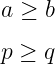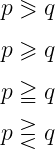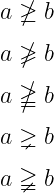# How to write greater than or equal to(≥) symbol in LaTeX?

Mathematically, there are different inequalities symbols to represent “greater than or equal to”. However, the ≥ symbol is used in 99 percent of cases. And there is a default \geq command for this symbol.

 Symbol Greater than or equal to Type Inequalities Package asmmath, mathabx Command \geq, \geqslant, …,etc Example \geq → ≥
\documentclass{article}
\begin{document}
$$a \geq b$$
$$p \geq q$$
\end{document}

Output :However, for other symbols, you need to use the amsmath and mathabx packages. For example

\documentclass{article}
\usepackage{amsmath,mathabx}
\begin{document}
$$p \eqslantgtr q$$
$$p \geqslant q$$
$$p \geqq q$$
$$p \gtreqless q$$
\end{document}

Output :And you can use not symbol with each of the above symbols.

\documentclass{article}
\usepackage{amsmath,mathabx}
\begin{document}
$$a \ngeq b$$
$$a \ngeqslant b$$
$$a \ngeqq b$$
$$a \gneq b$$
$$a \gneqq b$$
\end{document}

Output :With the help of latex, the command of all the symbols related to greater than or equal to is explained in this tutorial. I hope, you have found the answer to your question.#### Md Jidan Mondal

LaTeX expert with over 10 years of experience in document preparation and typesetting. Specializes in creating professional documents, reports, and presentations using LaTeX.